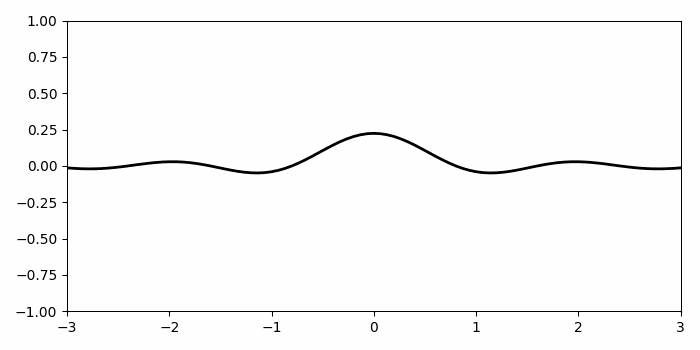# How to animate a line plot in Matplotlib?

To animate the line plot in matplotlib, we can take the following steps −

• Create a figure and a set of subplots using subplots() method.

• Limit x and y axes scale.

• Create x and t data points using numpy.

• Return coordinate matrices from coordinate vectors, X2 and T2.

• Plot a line with x and F data points using plot() method.

• To make animation plot, update y data.

• Make an animation by repeatedly calling a function *func*, current fig, animate, and interval.

• To display the figure, use show() method.

## Example

import numpy as np
from matplotlib import pyplot as plt, animation
plt.rcParams["figure.figsize"] = [7.00, 3.50]
plt.rcParams["figure.autolayout"] = True
fig, ax = plt.subplots()
ax.set(xlim=(-3, 3), ylim=(-1, 1))
x = np.linspace(-3, 3, 91)
t = np.linspace(1, 25, 30)
X2, T2 = np.meshgrid(x, t)
sinT2 = np.sin(2 * np.pi * T2 / T2.max())
F = 0.9 * sinT2 * np.sinc(X2 * (1 + sinT2))
line, = ax.plot(x, F[0, :], color='k', lw=2)
def animate(i):
line.set_ydata(F[i, :])
anim = animation.FuncAnimation(fig, animate, interval=100, frames=len(t) - 1)
anim.save('503.gif')
plt.show()

## Output

When we execute this code, it will display a line plot with animation.Updated on: 28-May-2021

2K+ Views